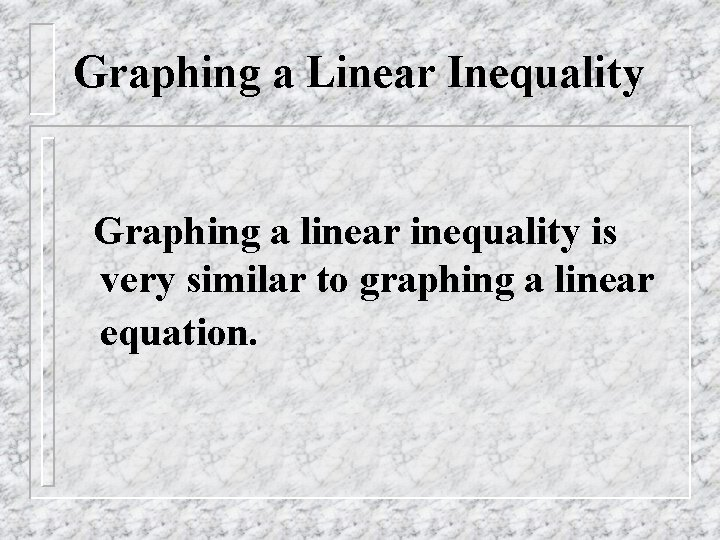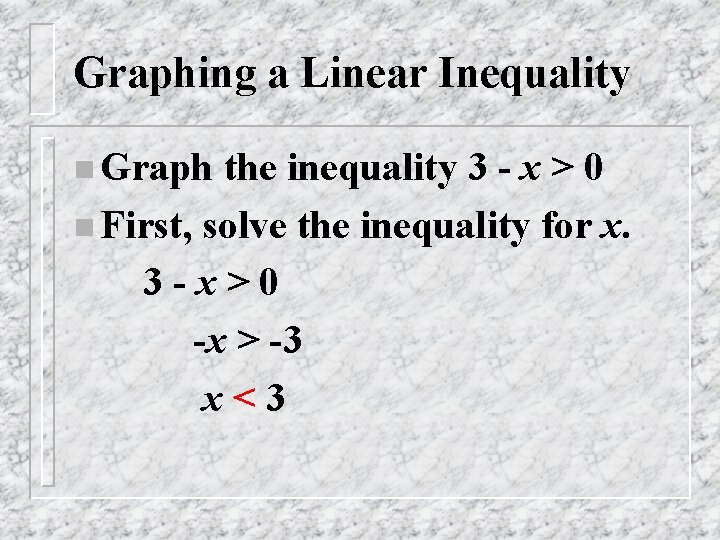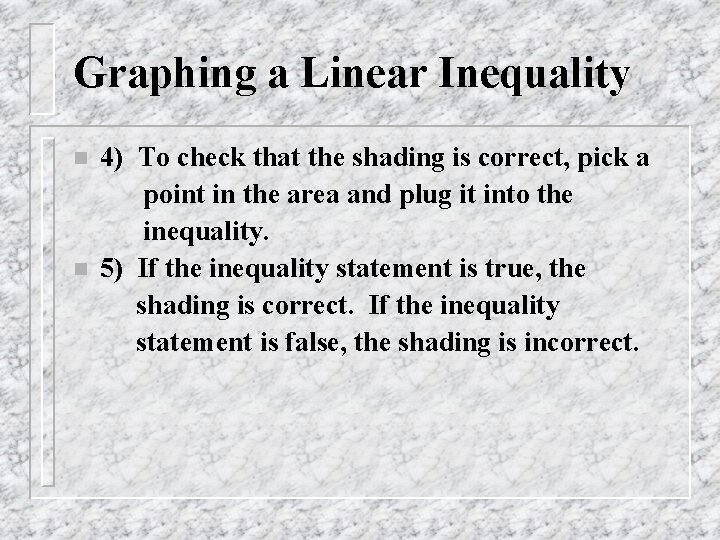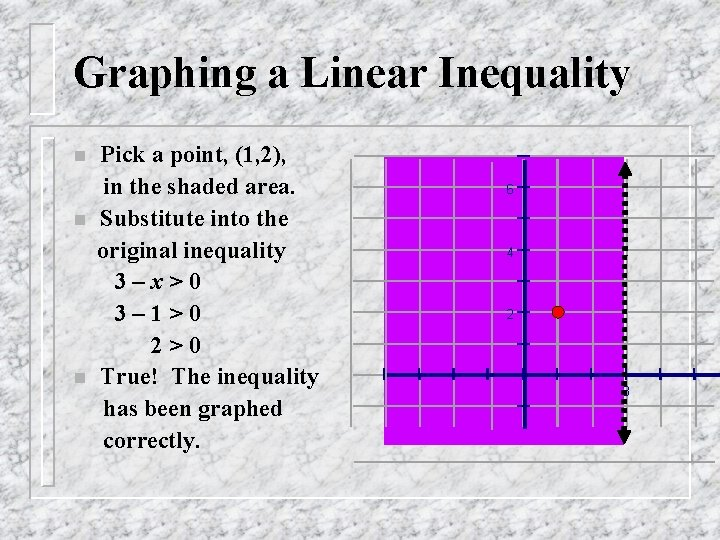Graphing a Linear Inequality Graphing a linear inequality

• Slides: 7Graphing a Linear Inequality Graphing a linear inequality is very similar to graphing a linear equation.Graphing a Linear Inequality 1) Solve the inequality for y (or for x if there is no y). n 2) Change the inequality to an equation and graph. n 3) If the inequality is < or >, the line is dotted. If the inequality is ≤ or ≥, the line is solid. nGraphing a Linear Inequality n Graph the inequality 3 - x > 0 n First, solve the inequality for x. 3 -x>0 -x > -3 x<3Graph: x<3 n Graph the line x = 3. 6 n Because x < 3 and not x ≤ 3, the line will be dotted. 4 2 n Now shade the side of the line where x < 3 (to the left of the line). 3Graphing a Linear Inequality n n 4) To check that the shading is correct, pick a point in the area and plug it into the inequality. 5) If the inequality statement is true, the shading is correct. If the inequality statement is false, the shading is incorrect.Graphing a Linear Inequality n n n Pick a point, (1, 2), in the shaded area. Substitute into the original inequality 3–x>0 3– 1>0 2>0 True! The inequality has been graphed correctly. 6 4 2 3Graphing Linear Inequalities using the Casio Calculator Go to the graph menu (5) n Delete all equations. n Before you enter your inequality, hit Type (F 3), then F 6. n Hit F 1 - F 4 to choose your inequality. n Now type in your inequality. n Hit F 6 to graph. n